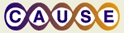# Teaching with Data SimulationsThis material was originally developed through CAUSE
as part of its collaboration with the SERC Pedagogic Service.

Researchers and educators have found that statistical ideas are often misunderstood by students and professionals. In order to develop better statistical reasoning, students need to first construct a deeper understanding of fundamental concepts. - delMas et al., 1999

## What is Teaching with Data Simulations?

Teaching with data simulations means giving students opportunities to simulate data in order to answer a particular research question or solve a statistical problem. There are several ways to use simulations: physical simulations of a process (e.g., taking repeated samples of Reese's pieces candies to simulate a sampling distribution for the proportion of orange candies), simulating a game or situation to estimate the chances of certain outcomes (e.g., playing Let's Make a Deal to estimate the chances of winning using two different strategies, to determine which is the better strategy), using probability models to simulate data to estimate the chance of a particular outcome (e.g., the chance of getting three of a kind when dealing five cards), or simulating data while varying parameters to illustrate a concept or deepen students' understanding of a process (e.g., simulating confidence intervals from different populations while varying sample size, level of confidence, or standard deviation). Another use of simulation is to generate data under a certain theory to test whether a particular outcome is surprising (e.g., if a student correctly identifies 8 out of 10 samples of cola correctly in a blind taste test, determining if this is just due to chance/guessing by simulating data based on what could be expected if the person is guessing and comparing their result to a simulated sampling distribution). Learn more here

## Why Teach using Data Simulations?

There are many reasons to use data simulation in the classroom. First, simulation is an important tool used by statisticians to solve problems, so students need to learn how to use simulation as a statistical problem solving tool. Second, simulating data can help students visualize and build a deep understanding of difficult and abstract statistical concepts, and to see dynamic processes, rather than static figures and illustrations. Third, simulations provide students a way to informally address questions involving statistical inference, before formally studying this topic later in a class. Fourth, simulations provide a way to actively engage students in making and testing conjectures about data, developing their reasoning about statistical concepts and procedures. Learn more here

## How to Teach using Data Simulations

Research that examines the use of simulations on student outcomes suggests that even 'well-designed' simulations are unlikely to be effective if the interaction with the student isn't carefully structured (Lane & Peres, 2006). Consequently, how simulations are used is of great importance. Simulations can involve physical materials (drawing items from a bag, tossing coins, sampling candies) or involve generating data on the computer (drawing samples from a population or generating data based on a probability model). Even when using computer simulations, Chance and Rossman (2006) suggest always beginning with a concrete simulation (e.g., having students take random samples of words from the Gettysburg address before taking simulated samples using their Sampling Words applet). Learn more here

## Classroom Examples

The Example Collectioncontains a growing number of data simulation activities. Each activity includes a complete list of materials, instructions, teaching tips, assessment ideas, and references.

Noteworthy activities include:

Coke vs. Pepsi Taste Test: Experiments and Inference about Cause

The Reese's Pieces Activity

Example Collection

## References on Teaching with Data Simulations

For print and web references relating to teaching statistics with data simulations References.

For information on available technologies for data simulation Available Technologies